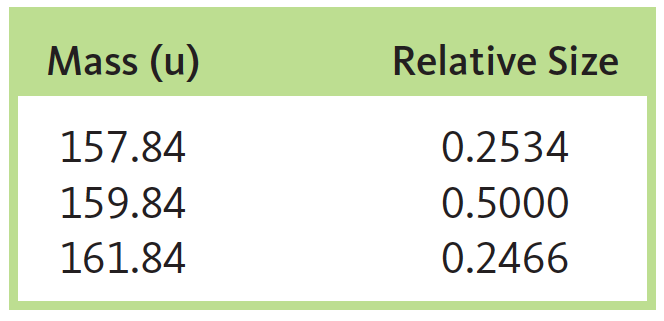# Problem: The mass spectrum of bromine (Br2) consists of three peaks with the following characteristics:How do you interpret these data?

###### FREE Expert Solution

Mass Spectrometry allows you to determine the molecular weight of an unknown compound through its vaporization and ionization.

Mass spectrometer is used to determine the mass to charge ratio of an unknown compound.

Divide the given masses into two to determine the possible isotopes of Br:

Peak 1: m Br2 = 157.84

m Br = 157.84/2

m1 Br = 78.92

Peak 2: m Br2 = 159.84

m Br = 159.84/2

m2 Br = 79.92

Peak 3: m Br2 = 161.84

m Br = 161.84/2

m3 Br = 80.92

From the spectrum, we can say that Br has 2 isotopes. One of the three peaks is a combination of the two isotopes. Knowing this, we can determine the isotopes:

m Br2 = m1 Br + m2 Br

m Br2 = 78.92 + 79.92

m Br2 = 158.84 → not in the peak (one of the mass is not an isotope)

89% (54 ratings)###### Problem Details

The mass spectrum of bromine (Br2) consists of three peaks with the following characteristics:How do you interpret these data?

Frequently Asked Questions

What scientific concept do you need to know in order to solve this problem?

Our tutors have indicated that to solve this problem you will need to apply the Mass Spectrometry concept. If you need more Mass Spectrometry practice, you can also practice Mass Spectrometry practice problems.

What professor is this problem relevant for?

Based on our data, we think this problem is relevant for Professor Staff's class at UGA.

What textbook is this problem found in?

Our data indicates that this problem or a close variation was asked in Chemistry: An Atoms First Approach - Zumdahl Atoms 1st 2nd Edition. You can also practice Chemistry: An Atoms First Approach - Zumdahl Atoms 1st 2nd Edition practice problems.